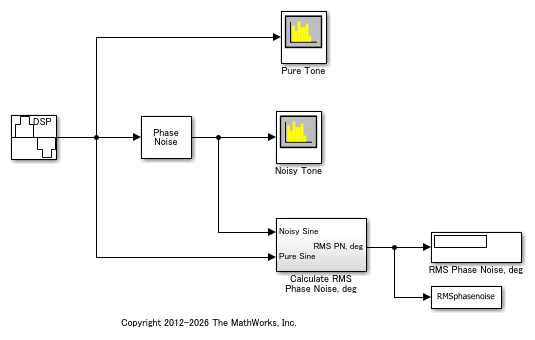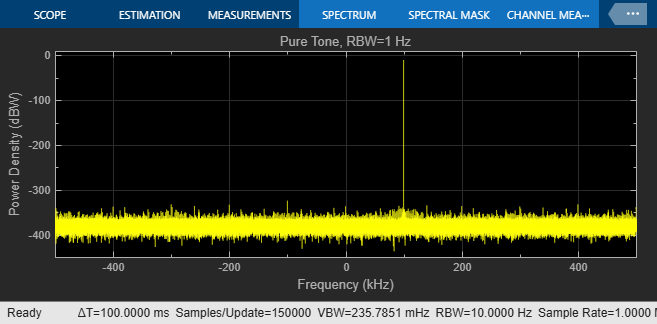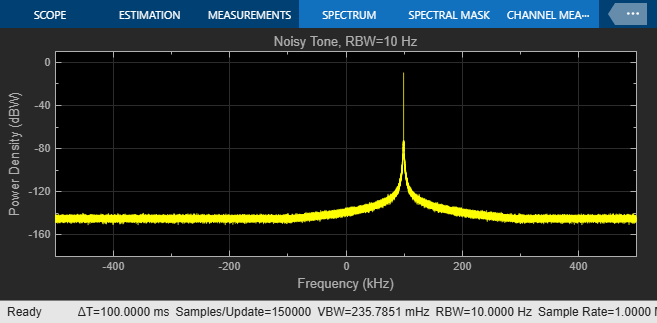# View Phase Noise Effects on Signal Spectrum in Simulink

This example shows the effects that spectral and phase noise have on a 100 kHz sine wave.

### Open Example Model and Explore Its ContentsThe `slex_phasenoise` model generates a 100 kHz tone by using a Sine Wave block and adds phase noise at these frequencies by using a Phase Noise block.

• `-85` dBc/Hz at a frequency offset of `1e3` Hz

• `-118` dBc/Hz at a frequency offset of `9.5e3` Hz

• `-125` dBc/Hz at a frequency offset of `19.5e3` Hz

• `-145` dBc/Hz at a frequency offset of `195e3` Hz

To analyze the spectrum and phase noise, the model includes three Spectrum Analyzer blocks configured for the `dBW/Hz` view:

• Pure Tone, RBW=1 Hz — 1 Hz resolution bandwidth

• Noisy Tone, RBW=1 Hz — 1 Hz resolution bandwidth

• Noisy Tone, RBW=10 Hz — 10 Hz resolution bandwidth

The model also includes a subsystem to calculate the RMS phase noise. The subsystem that calculates the RMS phase noise finds the phase error between the pure and noisy sine waves, then calculates the RMS phase noise in degrees. In general, to accurately determine the phase error, the pure signal must be time aligned with the noisy signal. However, the periodicity of the sine wave in this model makes this step unnecessary.

### Run the Model to Generate Results

The model displays the signal specturm and outputs the average of the calculated phase noise. The calculated phase noise achieves the spectrum defined by the Phase Noise block.

With the resolution bandwidth set to 1 Hz, the `dBW/Hz` view for the spectrum analyzer shows the tone at 0 dBW/Hz. With the resolution bandwidth set to 10 Hz, the `dBW/Hz` view for the spectrum analyzer shows the tone at -10 dBW/Hz. That same tone energy is now spread across 10 Hz instead of 1 Hz, so the sine wave PSD level reduces by 10 dB. With the resolution bandwidth at 10 Hz, the displayed average of the calculated phase noise still achieves the phase noise defined by the Phase Noise block.

The Spectrum Analyzer block achieves better spectral averaging with the wider resolution bandwidth. For more information, see Windows.

```The calculated average phase noise is 0.37 degrees. ```### Further Exploration

In the Phase Noise block, change the Phase noise level (dBc/Hz) parameter, rerun the model, and notice how the spectrum shape changes. With more noise, the side lobes increase in amplitude. As more phase noise is added, the 100 Hz signal becomes less distinct and the measured RMS phase noise increases.